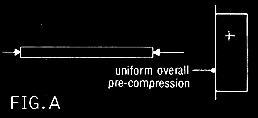Foundation, Concrete and Earthquake Engineering

### Positioning of the Prestressing Force

Application of compressive force to those zones of the concrete member where it is ultimately subjected to tensile stresses under working load conditions before the application of working loads, is the principle of prestressing. The relative less strength of concrete in tension with respect to that in compression, makes use of concrete conservative which is overcome by prestressing. The tensile stresses developed by these working loads are balanced by induced compressive strength.

Prestressing can be used to best advantage by varying the position of the prestress force. When the prestress is applied on the centroid of the cross-section a uniform compression is obtained. Consider the stress state of the beam in Fig. (B).We can see that by applying a prestress of the right magnitude we can produce pre-compression equal and opposite to the tensile force in Fig.(B). Then by adding the stress blocks we get: i.e. zero stress towards the bottom fibres and twice the compressive stress towards the top fibres.Now apply the pre-compression force at 1/3 the beam depth above the bottom face. As well as the overall compression we now have a further compressive stress acting on the bottom fibre due to the moment of the eccentric prestress force about the neutral axis of the section.

Figures to explain the post are from FIG. (A) to FIG. (E)
We then find it is possible to achieve the same compression at the bottom fibre with only half the prestressing force. See Fig.(D). Adding now the stress blocks of Fig. (B) and (D) we find that the tensile stress in the bottom fibre is again negated whilst the final compressive stress in the top fibre is only half that of Fig. (C). See Fig.(E). Thus by varying the position of the compressive force we can reduce the prestress force required, reduce the concrete strength required and sometimes reduce the cross sectional area. Changes in cross sections such as using T or I or channel sections rather than rectangular sections can lead to further economies.